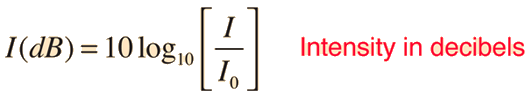# Logarithmic Time

So I had an interesting idea. For a project, A decibel is a unit of measurement used to express the ratio of one value of a power or field quantity to another, on a logarithmic scale. Instead of expressing the ratio of a value of power or field quantity use it to express time. I mean the standard equation is in the attacth image. I would want to replace I being Hours and I0 to be Minutes. This all just for fun.Permission granted.

Paul

You better not open a rift in the space-time continuum.

How would I go about writing a program like that? Frist, how would o program the arduino to do a logarithmic equation. Second, how could i used values from say ds3231 Real time clock module then have that figure displayed on like the serial monitor or LCD.

What do you want your program to do?

As you wrote, you need two values to compute a ratio. If the current time is one value, what is the other value?

Suggest you convert the times to seconds and then do the computation. Results are the same and the computation is much easier.

Paul

What I had in mind like nothing serious just to serve as amusement. I know very little about arduino I'm a novice. What I intended my program to do it use a ds3231 Real time clock module. To use the hour value divided by the minute value then, turn that into a logarithmic function. Like this Decibels but instead of the I reprenting sound intensity "I" replace it with hours and replace the standard threshold of hearing "I0" to minutes and then turn it in to a logarithmic expression. Output it in to an expression to the serial monitor or a simple 7 segment display. Now, I realize arduino uses vaules as expressed in like milisecond.

Look at the documentation for math.h:
http://www.cplusplus.com/reference/cmath/

As you can see, it includes natural log, log base 10 and log base 2 functions.

Intresting, Ill have to explore that.